# Package Modelica.​Blocks.​MathLibrary of Real mathematical functions as input/output blocks

### Information

This package contains basic mathematical operations, such as summation and multiplication, and basic mathematical functions, such as sqrt and sin, as input/output blocks. All blocks of this library can be either connected with continuous blocks or with sampled-data blocks.

Extends from `Modelica.​Icons.​Package` (Icon for standard packages).

### Package Contents

NameDescription
`Abs`Output the absolute value of the input
`Acos`Output the arc cosine of the input
`Add`Output the sum of the two inputs
`Add3`Output the sum of the three inputs
`Asin`Output the arc sine of the input
`Atan`Output the arc tangent of the input
`Atan2`Output atan(u1/u2) of the inputs u1 and u2
`BooleanChange`Indicates Boolean signal changing
`BooleanToInteger`Convert Boolean to Integer signal
`BooleanToReal`Convert Boolean to Real signal
`ContinuousMean`Calculates the empirical expectation (mean) value of its input signal
`Cos`Output the cosine of the input
`Cosh`Output the hyperbolic cosine of the input
`Division`Output first input divided by second input
`Edge`Indicates rising edge of Boolean signal
`Exp`Output the exponential (base e) of the input
`Feedback`Output difference between commanded and feedback input
`Gain`Output the product of a gain value with the input signal
`Harmonic`Calculate harmonic over period 1/f
`IntegerChange`Indicates integer signal changing
`IntegerToBoolean`Convert Integer to Boolean signal
`IntegerToReal`Convert Integer to Real signals
`InverseBlockConstraints`Construct inverse model by requiring that two inputs and two outputs are identical
`LinearDependency`Output a linear combination of the two inputs
`Log`Output the logarithm (default base e) of the input (input > 0 required)
`Log10`Output the base 10 logarithm of the input (input > 0 required)
`MatrixGain`Output the product of a gain matrix with the input signal vector
`Max`Pass through the largest signal
`Mean`Calculate mean over period 1/f
`Min`Pass through the smallest signal
`MinMax`Output the minimum and the maximum element of the input vector
`MultiProduct`Product of Reals: y = u*u* ... *u[n]
`MultiSum`Sum of Reals: y = k*u + k*u + ... + k[n]*u[n]
`MultiSwitch`Set Real expression that is associated with the first active input signal
`PolarToRectangular`Convert polar coordinates to rectangular coordinates
`Power`Output the power to a base of the input
`Product`Output product of the two inputs
`Pythagoras`Determines the hypotenuse or leg of a right triangle
`RealFFT`Sampling and FFT of input u
`RealToBoolean`Convert Real to Boolean signal
`RealToInteger`Convert Real to Integer signal
`RectangularToPolar`Convert rectangular coordinates to polar coordinates
`RectifiedMean`Calculate rectified mean over period 1/f
`RootMeanSquare`Calculate root mean square over period 1/f
`Sign`Output the sign of the input
`Sin`Output the sine of the input
`Sinh`Output the hyperbolic sine of the input
`Sqrt`Output the square root of the input (input >= 0 required)
`StandardDeviation`Calculates the empirical standard deviation of its input signal
`Sum`Output the sum of the elements of the input vector
`Tan`Output the tangent of the input
`Tanh`Output the hyperbolic tangent of the input
`TotalHarmonicDistortion`Output the total harmonic distortion (THD)
`UnitConversions`Conversion blocks to convert between SI and non-SI unit signals
`Variance`Calculates the empirical variance of its input signal
`WrapAngle`Wrap angle to interval ]-pi,pi] or [0,2*pi[

## Block Modelica.​Blocks.​Math.​InverseBlockConstraintsConstruct inverse model by requiring that two inputs and two outputs are identical

### Information

Exchange input and output signals of a block, i.e., the previous block inputs become block outputs and the previous block outputs become block inputs. This block is used to construct inverse models. Its usage is demonstrated in example: Modelica.Blocks.Examples.InverseModel.

Note, if a block shall be inverted that has several input and output blocks, then this can be easily achieved by using a vector of InverseBlockConstraints instances:

```   InverseBlockConstraint invert;  // Block to be inverted has 3 input signals
```

### Connectors

TypeNameDescription
`input ``RealInput``u1`Input signal 1 (u1 = u2)
`input ``RealInput``u2`Input signal 2 (u1 = u2)
`output ``RealOutput``y1`Output signal 1 (y1 = y2)
`output ``RealOutput``y2`Output signal 2 (y1 = y2)

## Block Modelica.​Blocks.​Math.​GainOutput the product of a gain value with the input signal

### Information

This block computes output y as product of gain k with the input u:

```    y = k * u;
```

### Parameters

TypeNameDefaultDescription
`Real``k` Gain value multiplied with input signal

### Connectors

TypeNameDescription
`input ``RealInput``u`Input signal connector
`output ``RealOutput``y`Output signal connector

## Block Modelica.​Blocks.​Math.​MatrixGainOutput the product of a gain matrix with the input signal vector

### Information

This blocks computes output vector y as product of the gain matrix K with the input signal vector u:

```    y = K * u;
```

Example:

```   parameter: K = [0.12 2; 3 1.5]

results in the following equations:

| y |     | 0.12  2.00 |   | u |
|      |  =  |            | * |      |
| y |     | 3.00  1.50 |   | u |
```

Extends from `Modelica.​Blocks.​Interfaces.​MIMO` (Multiple Input Multiple Output continuous control block).

### Parameters

TypeNameDefaultDescription
`Real``K[:,:]``[1,0; 0,1]`Gain matrix which is multiplied with the input
`final ``Integer``nin``size(K, 2)`Number of inputs
`final ``Integer``nout``size(K, 1)`Number of outputs

### Connectors

TypeNameDescription
`input ``RealInput``u[nin]`Connector of Real input signals
`output ``RealOutput``y[nout]`Connector of Real output signals

## Block Modelica.​Blocks.​Math.​MultiSumSum of Reals: y = k*u + k*u + ... + k[n]*u[n]

### Information

This blocks computes the scalar Real output "y" as sum of the elements of the Real input signal vector u:

```y = k*u + k*u + ... k[N]*u[N];
```

The input connector is a vector of Real input signals. When a connection line is drawn, the dimension of the input vector is enlarged by one and the connection is automatically connected to this new free index (thanks to the connectorSizing annotation).

The usage is demonstrated, e.g., in example Modelica.Blocks.Examples.RealNetwork1.

If no connection to the input connector "u" is present, the output is set to zero: y=0.

Extends from `Modelica.​Blocks.​Interfaces.​PartialRealMISO` (Partial block with a RealVectorInput and a RealOutput signal).

### Parameters

TypeNameDefaultDescription
`Integer``significantDigits``3`Number of significant digits to be shown in dynamic diagram layer for y
`Integer``nu``0`Number of input connections
`Real``k[nu]``fill(1, nu)`Input gains

### Connectors

TypeNameDescription
`input ``RealVectorInput``u[nu]`
`output ``RealOutput``y`

## Block Modelica.​Blocks.​Math.​MultiProductProduct of Reals: y = u*u* ... *u[n]

### Information

This blocks computes the scalar Real output "y" as product of the elements of the Real input signal vector u:

```y = u*u* ... *u[N];
```

The input connector is a vector of Real input signals. When a connection line is drawn, the dimension of the input vector is enlarged by one and the connection is automatically connected to this new free index (thanks to the connectorSizing annotation).

The usage is demonstrated, e.g., in example Modelica.Blocks.Examples.RealNetwork1.

If no connection to the input connector "u" is present, the output is set to zero: y=0.

Extends from `Modelica.​Blocks.​Interfaces.​PartialRealMISO` (Partial block with a RealVectorInput and a RealOutput signal).

### Parameters

TypeNameDefaultDescription
`Integer``significantDigits``3`Number of significant digits to be shown in dynamic diagram layer for y
`Integer``nu``0`Number of input connections

### Connectors

TypeNameDescription
`input ``RealVectorInput``u[nu]`
`output ``RealOutput``y`

## Block Modelica.​Blocks.​Math.​MultiSwitchSet Real expression that is associated with the first active input signal

### Information

This block has a vector of Boolean input signals u[nu] and a vector of (time varying) Real expressions expr[nu]. The output signal y is set to expr[i], if i is the first element in the input vector u that is true. If all input signals are false, y is set to parameter "y_default".

```  // Conceptual equation (not valid Modelica)
i = 'first element of u[:] that is true';
y = if i==0 then y_default else expr[i];
```

The input connector is a vector of Boolean input signals. When a connection line is drawn, the dimension of the input vector is enlarged by one and the connection is automatically connected to this new free index (thanks to the connectorSizing annotation).

The usage is demonstrated, e.g., in example Modelica.Blocks.Examples.RealNetwork1.

### Parameters

TypeNameDefaultDescription
`Real``y_default``0`Default value of output y if all u[i] = false
`Integer``nu``0`Number of input connections
`Integer``precision``3`Number of significant digits to be shown in dynamic diagram layer for y

### Connectors

TypeNameDescription
`input ``BooleanVectorInput``u[nu]`Set y = expr[i], if u[i] = true
`output ``RealOutput``y`Output depending on expression

## Block Modelica.​Blocks.​Math.​SumOutput the sum of the elements of the input vector

### Information

This blocks computes output y as sum of the elements of the input signal vector u:

```    y = u + u + ...;
```

Example:

```     parameter:   nin = 3;

results in the following equations:

y = u + u + u;
```

Extends from `Modelica.​Blocks.​Interfaces.​MISO` (Multiple Input Single Output continuous control block).

### Parameters

TypeNameDefaultDescription
`Integer``nin``1`Number of inputs
`Real``k[nin]``ones(nin)`Optional: sum coefficients

### Connectors

TypeNameDescription
`input ``RealInput``u[nin]`Connector of Real input signals
`output ``RealOutput``y`Connector of Real output signal

## Block Modelica.​Blocks.​Math.​FeedbackOutput difference between commanded and feedback input

### Information

This blocks computes output y as difference of the commanded input u1 and the feedback input u2:

```    y = u1 - u2;
```

Example:

```     parameter:   n = 2

results in the following equations:

y = u1 - u2
```

### Connectors

TypeNameDescription
`input ``RealInput``u1`
`input ``RealInput``u2`
`output ``RealOutput``y`

## Block Modelica.​Blocks.​Math.​AddOutput the sum of the two inputs

### Information

This blocks computes output y as sum of the two input signals u1 and u2:

```    y = k1*u1 + k2*u2;
```

Example:

```     parameter:   k1= +2, k2= -3

results in the following equations:

y = 2 * u1 - 3 * u2
```

Extends from `Modelica.​Blocks.​Interfaces.​SI2SO` (2 Single Input / 1 Single Output continuous control block).

### Parameters

TypeNameDefaultDescription
`Real``k1``1`Gain of input signal 1
`Real``k2``1`Gain of input signal 2

### Connectors

TypeNameDescription
`input ``RealInput``u1`Connector of Real input signal 1
`input ``RealInput``u2`Connector of Real input signal 2
`output ``RealOutput``y`Connector of Real output signal

## Block Modelica.​Blocks.​Math.​Add3Output the sum of the three inputs

### Information

This blocks computes output y as sum of the three input signals u1, u2 and u3:

```    y = k1*u1 + k2*u2 + k3*u3;
```

Example:

```     parameter:   k1= +2, k2= -3, k3=1;

results in the following equations:

y = 2 * u1 - 3 * u2 + u3;
```

Extends from `Modelica.​Blocks.​Icons.​Block` (Basic graphical layout of input/output block).

### Parameters

TypeNameDefaultDescription
`Real``k1``1`Gain of input signal 1
`Real``k2``1`Gain of input signal 2
`Real``k3``1`Gain of input signal 3

### Connectors

TypeNameDescription
`input ``RealInput``u1`Connector of Real input signal 1
`input ``RealInput``u2`Connector of Real input signal 2
`input ``RealInput``u3`Connector of Real input signal 3
`output ``RealOutput``y`Connector of Real output signal

## Block Modelica.​Blocks.​Math.​ProductOutput product of the two inputs

### Information

This blocks computes the output y as product of the two inputs u1 and u2:

```    y = u1 * u2;
```

Extends from `Modelica.​Blocks.​Interfaces.​SI2SO` (2 Single Input / 1 Single Output continuous control block).

### Connectors

TypeNameDescription
`input ``RealInput``u1`Connector of Real input signal 1
`input ``RealInput``u2`Connector of Real input signal 2
`output ``RealOutput``y`Connector of Real output signal

## Block Modelica.​Blocks.​Math.​DivisionOutput first input divided by second input

### Information

This block computes the output y by dividing the two inputs u1 and u2:

```    y = u1 / u2;
```

Extends from `Modelica.​Blocks.​Interfaces.​SI2SO` (2 Single Input / 1 Single Output continuous control block).

### Connectors

TypeNameDescription
`input ``RealInput``u1`Connector of Real input signal 1
`input ``RealInput``u2`Connector of Real input signal 2
`output ``RealOutput``y`Connector of Real output signal

## Block Modelica.​Blocks.​Math.​AbsOutput the absolute value of the input

### Information

This blocks computes the output y as absolute value of the input u:

```    y = abs( u );
```

The Boolean parameter generateEvent decides whether Events are generated at zero crossing (Modelica specification before 3) or not.

Extends from `Modelica.​Blocks.​Interfaces.​SISO` (Single Input Single Output continuous control block).

### Parameters

TypeNameDefaultDescription
`Boolean``generateEvent``false`Choose whether events shall be generated

### Connectors

TypeNameDescription
`input ``RealInput``u`Connector of Real input signal
`output ``RealOutput``y`Connector of Real output signal

## Block Modelica.​Blocks.​Math.​SignOutput the sign of the input

### Information

This blocks computes the output y as sign of the input u:

```         1  if u > 0
y =  0  if u == 0
-1  if u < 0
```

The Boolean parameter generateEvent decides whether Events are generated at zero crossing (Modelica specification before 3) or not.

Extends from `Modelica.​Blocks.​Interfaces.​SISO` (Single Input Single Output continuous control block).

### Parameters

TypeNameDefaultDescription
`Boolean``generateEvent``false`Choose whether events shall be generated

### Connectors

TypeNameDescription
`input ``RealInput``u`Connector of Real input signal
`output ``RealOutput``y`Connector of Real output signal

## Block Modelica.​Blocks.​Math.​SqrtOutput the square root of the input (input >= 0 required)

### Information

This blocks computes the output y as square root of the input u:

```    y = sqrt( u );
```

The input shall be zero or positive. Otherwise an error occurs.

Extends from `Modelica.​Blocks.​Interfaces.​SISO` (Single Input Single Output continuous control block).

### Connectors

TypeNameDescription
`input ``RealInput``u`Connector of Real input signal
`output ``RealOutput``y`Connector of Real output signal

## Block Modelica.​Blocks.​Math.​SinOutput the sine of the input

### Information

This blocks computes the output y as sine of the input u:

```    y = sin( u );
```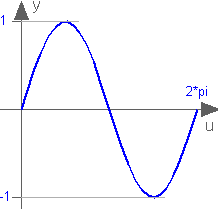Extends from `Modelica.​Blocks.​Interfaces.​SISO` (Single Input Single Output continuous control block).

### Connectors

TypeNameDescription
`input ``RealInput``u`Connector of Real input signal
`output ``RealOutput``y`Connector of Real output signal

## Block Modelica.​Blocks.​Math.​CosOutput the cosine of the input

### Information

This blocks computes the output y as cos of the input u:

```    y = cos( u );
```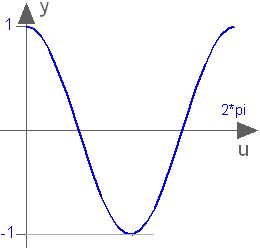Extends from `Modelica.​Blocks.​Interfaces.​SISO` (Single Input Single Output continuous control block).

### Connectors

TypeNameDescription
`input ``RealInput``u`Connector of Real input signal
`output ``RealOutput``y`Connector of Real output signal

## Block Modelica.​Blocks.​Math.​TanOutput the tangent of the input

### Information

This blocks computes the output y as tan of the input u:

```    y = tan( u );
```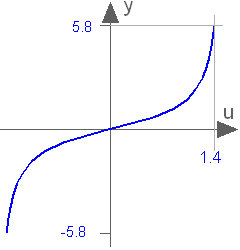Extends from `Modelica.​Blocks.​Interfaces.​SISO` (Single Input Single Output continuous control block).

### Connectors

TypeNameDescription
`input ``RealInput``u`Connector of Real input signal
`output ``RealOutput``y`Connector of Real output signal

## Block Modelica.​Blocks.​Math.​AsinOutput the arc sine of the input

### Information

This blocks computes the output y as the sine-inverse of the input u:

```    y = asin( u );
```

The absolute value of the input u need to be less or equal to one (abs( u ) <= 1). Otherwise an error occurs.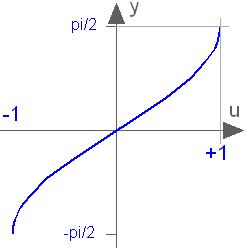Extends from `Modelica.​Blocks.​Interfaces.​SISO` (Single Input Single Output continuous control block).

### Connectors

TypeNameDescription
`input ``RealInput``u`Connector of Real input signal
`output ``RealOutput``y`Connector of Real output signal

## Block Modelica.​Blocks.​Math.​AcosOutput the arc cosine of the input

### Information

This blocks computes the output y as the cosine-inverse of the input u:

```    y = acos( u );
```

The absolute value of the input u need to be less or equal to one (abs( u ) <= 1). Otherwise an error occurs.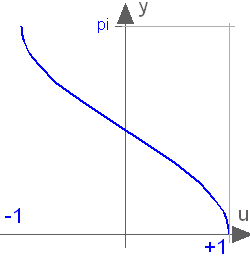Extends from `Modelica.​Blocks.​Interfaces.​SISO` (Single Input Single Output continuous control block).

### Connectors

TypeNameDescription
`input ``RealInput``u`Connector of Real input signal
`output ``RealOutput``y`Connector of Real output signal

## Block Modelica.​Blocks.​Math.​AtanOutput the arc tangent of the input

### Information

This blocks computes the output y as the tangent-inverse of the input u:

```    y= atan( u );
```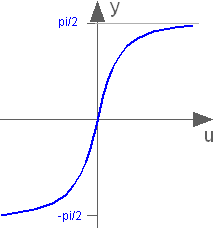Extends from `Modelica.​Blocks.​Interfaces.​SISO` (Single Input Single Output continuous control block).

### Connectors

TypeNameDescription
`input ``RealInput``u`Connector of Real input signal
`output ``RealOutput``y`Connector of Real output signal

## Block Modelica.​Blocks.​Math.​Atan2Output atan(u1/u2) of the inputs u1 and u2

### Information

This blocks computes the output y as the tangent-inverse of the input u1 divided by input u2:

```    y = atan2( u1, u2 );
```

u1 and u2 shall not be zero at the same time instant. Atan2 uses the sign of u1 and u2 in order to construct the solution in the range -180 deg ≤ y ≤ 180 deg, whereas block Atan gives a solution in the range -90 deg ≤ y ≤ 90 deg.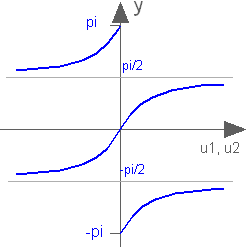Extends from `Modelica.​Blocks.​Interfaces.​SI2SO` (2 Single Input / 1 Single Output continuous control block).

### Connectors

TypeNameDescription
`input ``RealInput``u1`Connector of Real input signal 1
`input ``RealInput``u2`Connector of Real input signal 2
`output ``RealOutput``y`Connector of Real output signal

## Block Modelica.​Blocks.​Math.​SinhOutput the hyperbolic sine of the input

### Information

This blocks computes the output y as the hyperbolic sine of the input u:

```    y = sinh( u );
```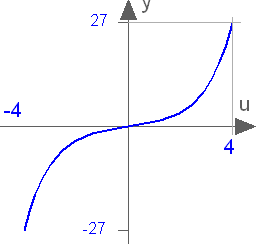Extends from `Modelica.​Blocks.​Interfaces.​SISO` (Single Input Single Output continuous control block).

### Connectors

TypeNameDescription
`input ``RealInput``u`Connector of Real input signal
`output ``RealOutput``y`Connector of Real output signal

## Block Modelica.​Blocks.​Math.​CoshOutput the hyperbolic cosine of the input

### Information

This blocks computes the output y as the hyperbolic cosine of the input u:

```    y = cosh( u );
```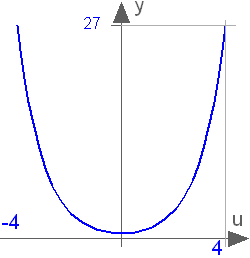Extends from `Modelica.​Blocks.​Interfaces.​SISO` (Single Input Single Output continuous control block).

### Connectors

TypeNameDescription
`input ``RealInput``u`Connector of Real input signal
`output ``RealOutput``y`Connector of Real output signal

## Block Modelica.​Blocks.​Math.​TanhOutput the hyperbolic tangent of the input

### Information

This blocks computes the output y as the hyperbolic tangent of the input u:

```    y = tanh( u );
```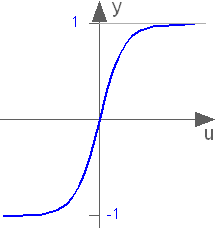Extends from `Modelica.​Blocks.​Interfaces.​SISO` (Single Input Single Output continuous control block).

### Connectors

TypeNameDescription
`input ``RealInput``u`Connector of Real input signal
`output ``RealOutput``y`Connector of Real output signal

## Block Modelica.​Blocks.​Math.​ExpOutput the exponential (base e) of the input

### Information

This blocks computes the output y as the exponential (of base e) of the input u:

```    y = exp( u );
```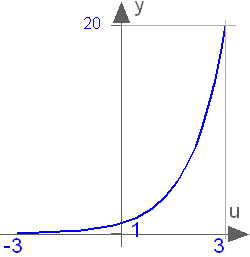Extends from `Modelica.​Blocks.​Interfaces.​SISO` (Single Input Single Output continuous control block).

### Connectors

TypeNameDescription
`input ``RealInput``u`Connector of Real input signal
`output ``RealOutput``y`Connector of Real output signal

## Block Modelica.​Blocks.​Math.​PowerOutput the power to a base of the input

### Information

This blocks computes the output y as the power to the parameter base of the input u. If the boolean parameter useExp is true, the output is determined by:

```    y = exp ( u * log (base) )
```

otherwise:

```    y = base ^ u;
```

Extends from `Modelica.​Blocks.​Interfaces.​SISO` (Single Input Single Output continuous control block).

### Parameters

TypeNameDefaultDescription
`Real``base``Modelica.​Constants.​e`Base of power
`Boolean``useExp``true`Use exp function in implementation

### Connectors

TypeNameDescription
`input ``RealInput``u`Connector of Real input signal
`output ``RealOutput``y`Connector of Real output signal

## Block Modelica.​Blocks.​Math.​LogOutput the logarithm (default base e) of the input (input > 0 required)

### Information

This blocks computes the output y as the logarithm to the parameter base of the input u:

```    y = log( u ) / log( base );
```

An error occurs if the input u is zero or negative.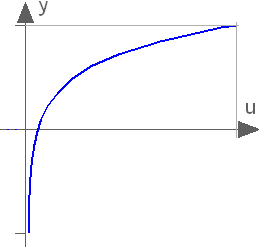Extends from `Modelica.​Blocks.​Interfaces.​SISO` (Single Input Single Output continuous control block).

### Parameters

TypeNameDefaultDescription
`Real``base``Modelica.​Constants.​e`Base of logarithm

### Connectors

TypeNameDescription
`input ``RealInput``u`Connector of Real input signal
`output ``RealOutput``y`Connector of Real output signal

## Block Modelica.​Blocks.​Math.​Log10Output the base 10 logarithm of the input (input > 0 required)

### Information

This blocks computes the output y as the base 10 logarithm of the input u:

```    y = log10( u );
```

An error occurs if the input u is zero or negative.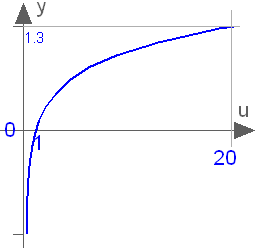Extends from `Modelica.​Blocks.​Interfaces.​SISO` (Single Input Single Output continuous control block).

### Connectors

TypeNameDescription
`input ``RealInput``u`Connector of Real input signal
`output ``RealOutput``y`Connector of Real output signal

## Block Modelica.​Blocks.​Math.​WrapAngleWrap angle to interval ]-pi,pi] or [0,2*pi[

### Information

This blocks wraps the input angle into the interval ]-pi,pi], if `positiveRange == false`. Otherwise the input angle `u` is wrapped to the interval [0,2*pi[.

Extends from `Modelica.​Blocks.​Interfaces.​SISO` (Single Input Single Output continuous control block).

### Parameters

TypeNameDefaultDescription
`Boolean``positiveRange``false`Use only positive output range, if true

### Connectors

TypeNameDescription
`input ``RealInput``u`Connector of Real input signal
`output ``RealOutput``y`Connector of Real output signal

## Block Modelica.​Blocks.​Math.​RealToIntegerConvert Real to Integer signal

### Information

This block computes the output y as nearest integer value of the input u:

```    y = integer( floor( u + 0.5 ) )  for  u > 0;
y = integer( ceil ( u - 0.5 ) )  for  u < 0;
```

Extends from `Modelica.​Blocks.​Icons.​IntegerBlock` (Basic graphical layout of Integer block).

### Connectors

TypeNameDescription
`input ``RealInput``u`Connector of Real input signal
`output ``IntegerOutput``y`Connector of Integer output signal

## Block Modelica.​Blocks.​Math.​IntegerToRealConvert Integer to Real signals

### Information

This block computes the output y as Real equivalent of the Integer input u:

```    y = u;
```

where u is of Integer and y of Real type.

Extends from `Modelica.​Blocks.​Icons.​Block` (Basic graphical layout of input/output block).

### Connectors

TypeNameDescription
`input ``IntegerInput``u`Connector of Integer input signal
`output ``RealOutput``y`Connector of Real output signal

## Block Modelica.​Blocks.​Math.​BooleanToRealConvert Boolean to Real signal

### Information

This block computes the output y as Real equivalent of the Boolean input u:

```    y = if u then realTrue else realFalse;
```

where u is of Boolean and y of Real type, and realTrue and realFalse are parameters.

Extends from `Modelica.​Blocks.​Interfaces.​partialBooleanSI` (Partial block with 1 input Boolean signal).

### Parameters

TypeNameDefaultDescription
`Real``realTrue``1`Output signal for true Boolean input
`Real``realFalse``0`Output signal for false Boolean input

### Connectors

TypeNameDescription
`input ``BooleanInput``u`Connector of Boolean input signal
`output ``RealOutput``y`Connector of Real output signal

## Block Modelica.​Blocks.​Math.​BooleanToIntegerConvert Boolean to Integer signal

### Information

This block computes the output y as Integer equivalent of the Boolean input u:

```    y = if u then integerTrue else integerFalse;
```

where u is of Boolean and y of Integer type, and integerTrue and integerFalse are parameters.

Extends from `Modelica.​Blocks.​Interfaces.​partialBooleanSI` (Partial block with 1 input Boolean signal).

### Parameters

TypeNameDefaultDescription
`Integer``integerTrue``1`Output signal for true Boolean input
`Integer``integerFalse``0`Output signal for false Boolean input

### Connectors

TypeNameDescription
`input ``BooleanInput``u`Connector of Boolean input signal
`output ``IntegerOutput``y`Connector of Integer output signal

## Block Modelica.​Blocks.​Math.​RealToBooleanConvert Real to Boolean signal

### Information

This block computes the Boolean output y from the Real input u by the equation:

```    y = u ≥ threshold;
```

where threshold is a parameter.

Extends from `Modelica.​Blocks.​Interfaces.​partialBooleanSO` (Partial block with 1 output Boolean signal).

### Parameters

TypeNameDefaultDescription
`Real``threshold``0.5`Output signal y is true, if input u >= threshold

### Connectors

TypeNameDescription
`input ``RealInput``u`Connector of Real input signal
`output ``BooleanOutput``y`Connector of Boolean output signal

## Block Modelica.​Blocks.​Math.​IntegerToBooleanConvert Integer to Boolean signal

### Information

This block computes the Boolean output y from the Integer input u by the equation:

```    y = u ≥ threshold;
```

where threshold is a parameter.

Extends from `Modelica.​Blocks.​Interfaces.​partialBooleanSO` (Partial block with 1 output Boolean signal).

### Parameters

TypeNameDefaultDescription
`Integer``threshold``1`Output signal y is true, if input u >= threshold

### Connectors

TypeNameDescription
`input ``IntegerInput``u`Connector of Integer input signal
`output ``BooleanOutput``y`Connector of Boolean output signal

## Block Modelica.​Blocks.​Math.​RectangularToPolarConvert rectangular coordinates to polar coordinates

### Information

The input values of this block are the rectangular components `u_re` and `u_im` of a phasor in two dimensions. This block calculates the length `y_abs` and the angle `y_arg` of the polar representation of this phasor.

```  y_abs = abs(u_re + j*u_im) = sqrt( u_re2 + u_im2 )
y_arg = arg(u_re + j*u_im) = atan2(u_im, u_re)
```

Extends from `Modelica.​Blocks.​Icons.​Block` (Basic graphical layout of input/output block).

### Connectors

TypeNameDescription
`input ``RealInput``u_re`Real part of rectangular representation
`input ``RealInput``u_im`Imaginary part of rectangular representation
`output ``RealOutput``y_abs`Length of polar representation
`output ``RealOutput``y_arg`Angle of polar representation

## Block Modelica.​Blocks.​Math.​PolarToRectangularConvert polar coordinates to rectangular coordinates

### Information

The input values of this block are the polar components `uabs` and `uarg` of a phasor. This block calculates the components `y_re` and `y_im` of the rectangular representation of this phasor.

```   y_re = u_abs * cos( u_arg )
y_im = u_abs * sin( u_arg )
```

Extends from `Modelica.​Blocks.​Icons.​Block` (Basic graphical layout of input/output block).

### Connectors

TypeNameDescription
`input ``RealInput``u_abs`Length of polar representation
`input ``RealInput``u_arg`Angle of polar representation
`output ``RealOutput``y_re`Real part of rectangular representation
`output ``RealOutput``y_im`Imaginary part of rectangular representation

## Block Modelica.​Blocks.​Math.​MeanCalculate mean over period 1/f

### Information

This block calculates the mean of the input signal u over the given period 1/f:

```1 T
- ∫ u(t) dt
T 0
```

Note: The output is updated after each period defined by 1/f.

If parameter yGreaterOrEqualZero in the Advanced tab is true (default = false), then the modeller provides the information that the mean of the input signal is guaranteed to be ≥ 0 for the exact solution. However, due to inaccuracies in the numerical integration scheme, the output might be slightly negative. If this parameter is set to true, then the output is explicitly set to 0.0, if the mean value results in a negative value.

Extends from `Modelica.​Blocks.​Interfaces.​SISO` (Single Input Single Output continuous control block).

### Parameters

TypeNameDefaultDescription
`Frequency``f` Base frequency
`Real``x0``0`Start value of integrator state
`Boolean``yGreaterOrEqualZero``false`=true, if output y is guaranteed to be >= 0 for the exact solution

### Connectors

TypeNameDescription
`input ``RealInput``u`Connector of Real input signal
`output ``RealOutput``y`Connector of Real output signal

## Block Modelica.​Blocks.​Math.​RectifiedMeanCalculate rectified mean over period 1/f

### Information

This block calculates the rectified mean of the input signal u over the given period 1/f, using the mean block.

Note: The output is updated after each period defined by 1/f.

Extends from `Modelica.​Blocks.​Interfaces.​SISO` (Single Input Single Output continuous control block).

### Parameters

TypeNameDefaultDescription
`Frequency``f` Base frequency
`Real``x0``0`Start value of integrator state

### Connectors

TypeNameDescription
`input ``RealInput``u`Connector of Real input signal
`output ``RealOutput``y`Connector of Real output signal

## Block Modelica.​Blocks.​Math.​ContinuousMeanCalculates the empirical expectation (mean) value of its input signal

### Information

This block continuously calculates the mean value of its input signal. It uses the function:

```    integral( u over time)
y = ----------------------
time - startTime```

This can be used to determine the empirical expectation value of a random signal, such as generated by the Noise blocks.

The parameter t_eps is used to guard against division by zero (the mean value computation starts at <simulation start time> + t_eps and before that time instant y = u).

This block is demonstrated in the examples UniformNoiseProperties and NormalNoiseProperties.

Extends from `Modelica.​Blocks.​Icons.​Block` (Basic graphical layout of input/output block).

### Parameters

TypeNameDefaultDescription
`Time``t_eps``1e-7`Mean value calculation starts at startTime + t_eps

### Connectors

TypeNameDescription
`input ``RealInput``u`Noisy input signal
`output ``RealOutput``y`Expectation (mean) value of the input signal

## Block Modelica.​Blocks.​Math.​RootMeanSquareCalculate root mean square over period 1/f

### Information

This block calculates the root mean square of the input signal u over the given period 1/f, using the mean block.

Note: The output is updated after each period defined by 1/f.

Extends from `Modelica.​Blocks.​Interfaces.​SISO` (Single Input Single Output continuous control block).

### Parameters

TypeNameDefaultDescription
`Frequency``f` Base frequency
`Real``x0``0`Start value of integrator state

### Connectors

TypeNameDescription
`input ``RealInput``u`Connector of Real input signal
`output ``RealOutput``y`Connector of Real output signal

## Block Modelica.​Blocks.​Math.​VarianceCalculates the empirical variance of its input signal

### Information

This block calculates the empirical variance of its input signal. It is based on the formula (but implemented in a more reliable numerical way):

`y = mean(  (u - mean(u))^2  )`

The parameter t_eps is used to guard against division by zero (the variance computation starts at <simulation start time> + t_eps and before that time instant y = 0).

The variance of a signal is also equal to its mean power.

This block is demonstrated in the examples UniformNoiseProperties and NormalNoiseProperties.

Extends from `Modelica.​Blocks.​Icons.​Block` (Basic graphical layout of input/output block).

### Parameters

TypeNameDefaultDescription
`Time``t_eps``1e-7`Variance calculation starts at startTime + t_eps

### Connectors

TypeNameDescription
`input ``RealInput``u`Noisy input signal
`output ``RealOutput``y`Variance of the input signal

## Block Modelica.​Blocks.​Math.​StandardDeviationCalculates the empirical standard deviation of its input signal

### Information

This block calculates the standard deviation of its input signal. The standard deviation is the square root of the signal's variance:

`y = sqrt( variance(u) )`

The Variance block is used to calculate variance(u).

The parameter t_eps is used to guard against division by zero (the computation of the standard deviation starts at <simulation start time> + t_eps and before that time instant y = 0).

This block is demonstrated in the examples UniformNoiseProperties and NormalNoiseProperties.

Extends from `Modelica.​Blocks.​Icons.​Block` (Basic graphical layout of input/output block).

### Parameters

TypeNameDefaultDescription
`Time``t_eps``1e-7`Standard deviation calculation starts at startTime + t_eps

### Connectors

TypeNameDescription
`input ``RealInput``u`Noisy input signal
`output ``RealOutput``y`Standard deviation of the input signal

## Block Modelica.​Blocks.​Math.​HarmonicCalculate harmonic over period 1/f

### Information

This block calculates the root mean square and the phase angle of a single harmonic k of the input signal u over the given period 1/f, using the mean block.

Note: The output is updated after each period defined by 1/f.

Note:
The harmonic is defined by `√2 rms cos(k 2 π f t - arg)` if useConjugateComplex=false (default)
The harmonic is defined by `√2 rms cos(k 2 π f t + arg)` if useConjugateComplex=true

Extends from `Modelica.​Blocks.​Icons.​Block` (Basic graphical layout of input/output block).

### Parameters

TypeNameDefaultDescription
`Frequency``f` Base frequency
`Integer``k` Order of harmonic
`Boolean``useConjugateComplex``false`Gives conjugate complex result if true
`Real``x0Cos``0`Start value of cos integrator state
`Real``x0Sin``0`Start value of sin integrator state

### Connectors

TypeNameDescription
`input ``RealInput``u`
`output ``RealOutput``y_rms`Root mean square of polar representation
`output ``RealOutput``y_arg`Angle of polar representation

## Block Modelica.​Blocks.​Math.​TotalHarmonicDistortionOutput the total harmonic distortion (THD)

### Information

This block determines the total harmonic distortion (THD) over the given period `1/f`. Consider that the input `u` consists of harmonic RMS components `U1`, `U2`, `U3`, etc. The total RMS component is then determined by: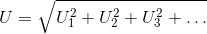The calculation of the total harmonic distortion is based on the parameter `useFirstHarmonic`. The default value `useFirstHarmonic = true` represents the standard THD calculation used in electrical engineering. The non-default value `useFirstHarmonic = false` calculates the THD typically used for the assessment of audio signals.

If `useFirstHarmonic = true`, the total higher harmonic content (harmonic order numbers > 1) refers to the RMS value of the fundamental wave: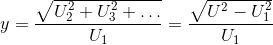If `useFirstHarmonic = false`, the total higher harmonic content (harmonic order numbers > 1) refers to the total RMS: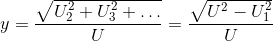In case of a zero input signal or within the first period of calculation, the boolean output signal `valid` becomes `false` to indicate that the calculation result is not valid. Valid calculations are indicated by `valid = true`.

Extends from `Modelica.​Blocks.​Interfaces.​SISO` (Single Input Single Output continuous control block).

### Parameters

TypeNameDefaultDescription
`Frequency``f` Base frequency
`Boolean``useFirstHarmonic``true`THD with respect to first harmonic, if true; otherwise with respect to total RMS

### Connectors

TypeNameDescription
`input ``RealInput``u`Connector of Real input signal
`output ``RealOutput``y`Connector of Real output signal
`output ``BooleanOutput``valid`True, if output y is valid

## Block Modelica.​Blocks.​Math.​RealFFTSampling and FFT of input u

### Information

This block samples the input signal, calculates the Fast Fourier Transform by function Math.realFFT, and (when simulation terminates) writes the output to result file resultFileName by function Math.realFFTwriteToFile.

The number of sampling points as well as the samplePeriod is calculated from desired maximum frequency f_max and frequency resolution f_res.

#### Note

The user has to take care that enough points can be sampled before the simulation ends: startTime + (ns - 1)*samplePeriod <= stopTime.

The result file is written as mat, first column = frequency, second column = amplitudes, third column = phases. The frequency points are separated by rows with amplitude and phase = 0, so one can plot the result directly as frequency lines.

Extends from `Modelica.​Blocks.​Interfaces.​DiscreteBlock` (Base class of discrete control blocks).

### Parameters

TypeNameDefaultDescription
`final ``Time``samplePeriod``(2 * f_res * div(ns, 2)) ^ (-1)`Sample period of component
`Time``startTime``0`First sample time instant
`Frequency``f_max` Maximum frequency of interest
`Frequency``f_res` Frequency resolution
`final ``Integer``ns``Modelica.Math.FastFourierTransform.realFFTsamplePoints(f_max, f_res, f_max_factor = 5)`Number of samples
`final ``Integer``nf``max(1, min(integer(ceil(f_max / f_res)) + 1, div(ns, 2)))`Number of frequency points
`String``resultFileName``"realFFT.mat"`Result file: f, abs, arg

### Connectors

TypeNameDescription
`input ``RealInput``u`

## Block Modelica.​Blocks.​Math.​PythagorasDetermines the hypotenuse or leg of a right triangle

### Information

This block determines the hypotenuse `y = sqrt(u1^2 + u2^2)` if the boolean parameter `u1IsHyotenuse = false`. In this case the two inputs `u1` and `u2` are interpreted as the legs of a right triangle and the boolean output `valid` is always equal to `true`.

If `u1IsHyotenuse = true`, input `u1` is interpreted as hypotenuse and `u2` is one of the two legs of a right triangle. Then, the other of the two legs of the right triangle is the output, determined by `y = sqrt(u1^2 - u2^2)`, if `u1^2 - u2^2 ≥ 0`; in this case the boolean output `valid` is equal to `true`. In case of `u1^2 - u2^2 < 0`, the output `y = 0` and `valid` is set to `false`.

Extends from `Modelica.​Blocks.​Interfaces.​SI2SO` (2 Single Input / 1 Single Output continuous control block).

### Parameters

TypeNameDefaultDescription
`Boolean``u1IsHypotenuse``false`If true, u1 is the hypotenuse and y is one leg

### Connectors

TypeNameDescription
`input ``RealInput``u1`Connector of Real input signal 1
`input ``RealInput``u2`Connector of Real input signal 2
`output ``RealOutput``y`Connector of Real output signal
`output ``BooleanOutput``valid`Is true, if y is a valid result

## Block Modelica.​Blocks.​Math.​MaxPass through the largest signal

### Information

This block computes the output y as maximum of the two Real inputs u1 and u2:

```    y = max ( u1 , u2 );
```

Extends from `Modelica.​Blocks.​Interfaces.​SI2SO` (2 Single Input / 1 Single Output continuous control block).

### Connectors

TypeNameDescription
`input ``RealInput``u1`Connector of Real input signal 1
`input ``RealInput``u2`Connector of Real input signal 2
`output ``RealOutput``y`Connector of Real output signal

## Block Modelica.​Blocks.​Math.​MinPass through the smallest signal

### Information

This block computes the output y as minimum of the two Real inputs u1 and u2:

```    y = min ( u1 , u2 );
```

Extends from `Modelica.​Blocks.​Interfaces.​SI2SO` (2 Single Input / 1 Single Output continuous control block).

### Connectors

TypeNameDescription
`input ``RealInput``u1`Connector of Real input signal 1
`input ``RealInput``u2`Connector of Real input signal 2
`output ``RealOutput``y`Connector of Real output signal

## Block Modelica.​Blocks.​Math.​MinMaxOutput the minimum and the maximum element of the input vector

### Information

Determines the minimum and maximum element of the input vector and provide both values as output.

Extends from `Modelica.​Blocks.​Icons.​Block` (Basic graphical layout of input/output block).

### Parameters

TypeNameDefaultDescription
`Integer``nu``0`Number of input connections

### Connectors

TypeNameDescription
`input ``RealVectorInput``u[nu]`
`output ``RealOutput``yMax`
`output ``RealOutput``yMin`

## Block Modelica.​Blocks.​Math.​LinearDependencyOutput a linear combination of the two inputs

### Information

Determine the linear combination of the two inputs: `y = y0*(1 + k1*u1 + k2*u2)`

Note, for y0=0 the output is always zero.

To improve the implementation, the formula will be changed (non-backwards compatible) in the future: `y = y0 + k1*u1 + k2*u2`

Extends from `Modelica.​Blocks.​Interfaces.​SI2SO` (2 Single Input / 1 Single Output continuous control block).

### Parameters

TypeNameDefaultDescription
`Real``y0``0`Initial value
`Real``k1``0`u1 dependency
`Real``k2``0`u2 dependency

### Connectors

TypeNameDescription
`input ``RealInput``u1`Connector of Real input signal 1
`input ``RealInput``u2`Connector of Real input signal 2
`output ``RealOutput``y`Connector of Real output signal

## Block Modelica.​Blocks.​Math.​EdgeIndicates rising edge of Boolean signal

### Information

This block sets the Boolean output y to true, when the Boolean input u shows a rising edge:

```    y = edge( u );
```

Extends from `Modelica.​Blocks.​Interfaces.​BooleanSISO` (Single Input Single Output control block with signals of type Boolean).

### Connectors

TypeNameDescription
`input ``BooleanInput``u`Connector of Boolean input signal
`output ``BooleanOutput``y`Connector of Boolean output signal

## Block Modelica.​Blocks.​Math.​BooleanChangeIndicates Boolean signal changing

### Information

This block sets the Boolean output y to true, when the Boolean input u shows a rising or falling edge, i.e., when the signal changes:

```    y = change( u );
```

Extends from `Modelica.​Blocks.​Interfaces.​BooleanSISO` (Single Input Single Output control block with signals of type Boolean).

### Connectors

TypeNameDescription
`input ``BooleanInput``u`Connector of Boolean input signal
`output ``BooleanOutput``y`Connector of Boolean output signal

## Block Modelica.​Blocks.​Math.​IntegerChangeIndicates integer signal changing

### Information

This block sets the Boolean output y to true, when the Integer input u changes:

```    y = change( u );
```

Extends from `Modelica.​Blocks.​Interfaces.​IntegerSIBooleanSO` (Integer Input Boolean Output continuous control block).

### Connectors

TypeNameDescription
`input ``IntegerInput``u`Connector of Integer input signal
`output ``BooleanOutput``y`Connector of Boolean output signal

Generated 2018-12-12 12:09:56 EST by MapleSim.